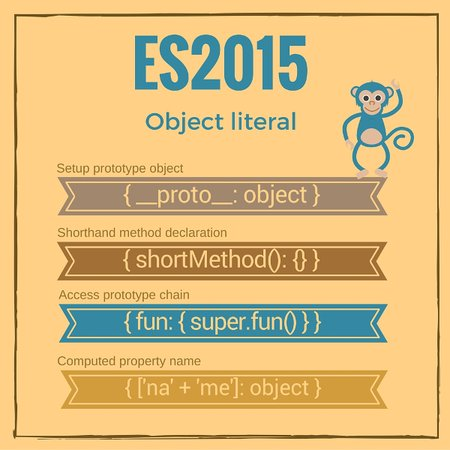Javascript 中的对象字面量很酷，你觉得呢？

ECMAScript 2015 之前，Javascript 中的对象字面量(又叫做对象初始化器)是相当简单的，它可以定义2种属性：

• 成对的静态属性名和值 `{ name1: value1 }`
• 通过 getters `{ get name(){..} }`setters `{ set name(val){..} }` 定义的动态计算属性值

``````    var myObject = {
myString: 'value 1',
get myNumber() {
return this.myNumber;
},
set myNumber(value) {
this.myNumber = Number(value);
}
};
myObject.myString; // => 'value 1'
myObject.myNumber = '15';
myObject.myNumber; // => 15
``````

JavaScript 是一种基于原型继承的语言，所以啥都是个对象。 所以当处理对象的创建、原型的设置与访问时，它必须提供简单的构造方法。

``````    var myProto = {
propertyExists: function(name) {
return name in this;
}
};
var myNumbers = Object.create(myProto);
myNumbers['array'] = [1, 6, 7];
myNumbers.propertyExists('array');      // => true
myNumbers.propertyExists('collection'); // => false
``````

• 在对象构造函数中设置原型
• 速写式方法声明
• 进行 `super` 调用
• 可计算的属性名1. 在对象构造函数中设置原型

``````    var myObject = {
name: 'Hello World!'
};
myObject.__proto__;                         // => {}
myObject.__proto__.isPrototypeOf(myObject); // => true

`myObject.__proto__` 返回 `myObject` 的原型对象。
``````

``````    var myProto = {
propertyExists: function(name) {
return name in this;
}
};
var myNumbers = {
__proto__: myProto,
array: [1, 6, 7]
};
myNumbers.propertyExists('array');      // => true
myNumbers.propertyExists('collection'); // => false
``````

`myNumbers` 是使用了特殊的属性名 `__proto__` 创建的对象，它的原型是 `myProto` 。 这个对象用了一个简单的声明来创建，没有使用类似 `Object.create()` 的附加函数。

• 让事情变得简单直接很复杂
• 让事情变得复杂难以理解很容易

1.1 特殊的情况下 `__proto__` 的使用手册``````    var object = {
__proto__: {
toString: function() {
return '[object Numbers]'
}
},
numbers: [1, 5, 89],
__proto__: {
toString: function() {
return '[object ArrayOfNumbers]'
}
}
};
``````

JavaScript 有只能使用对象或 `null` 作为 `__proto__` 属性值的约束。任何尝试使用原始类型们 (字符串，数字，布尔值) 乃至 `undefined` 会被忽略掉，不能改变对象的原型。 让我们看看这个限制的例子：

``````    var objUndefined = {
__proto__: undefined
};
Object.getPrototypeOf(objUndefined); // => {}
var objNumber = {
__proto__: 15
};
Object.getPrototypeOf(objNumber);    // => {}
``````

2. 速写式方法声明

``````    var collection = {
items: [],
this.items.push(item);
},
get(index) {
return this.items[index];
}
};
collection.get(0); // => 15
``````

`add()``get()``collection` 里用这个短模式定义的方法。

3. 进行 `super` 调用

``````    var calc = {
sumArray (items) {
return items.reduce(function(a, b) {
return a + b;
});
}
};
var numbers = {
__proto__: calc,
numbers: [4, 6, 7],
sumElements() {
return super.sumArray(this.numbers);
}
};
numbers.sumElements(); // => 17
``````

`calc``numbers` 对象的原型。在 `numbers``sumElements` 方法中可以通过 `super` 关键字调用原型的 `super.sumArray()` 方法。

3.1 `super` 的使用限制

`super` 在对象字面量中 只能在速写式方法声明里 使用。

``````    var calc = {
sumArray (items) {
return items.reduce(function(a, b) {
return a + b;
});
}
};
var numbers = {
__proto__: calc,
numbers: [4, 6, 7],
sumElements: function() {
return super.sumArray(this.numbers);
}
};
// Throws SyntaxError: 'super' keyword unexpected here
numbers.sumElements();
``````

4. 可计算的属性名

``````    function prefix(prefStr, name) {
return prefStr + '_' + name;
}
var object = {};
object[prefix('number', 'pi')] = 3.14;
object[prefix('bool', 'false')] = false;
object; // => { number_pi: 3.14, bool_false: false }
``````

``````    function prefix(prefStr, name) {
return prefStr + '_' + name;
}
var object = {
[prefix('number', 'pi')]: 3.14,
[prefix('bool', 'false')]: false
};
object; // => { number_pi: 3.14, bool_false: false }
``````

`[prefix('number', 'pi')]` 通过计算 `prefix('number', 'pi')` 表达式设置了 `'number_pi'` 这个属性名.

4.1 `Symbol` 作为属性名

Symbols 运算也可以作为可计算的属性名。只需要保证把它们括在括号里： `{ [Symbol('name')]: 'Prop value' }`

``````    var object = {
number1: 14,
number2: 15,
string1: 'hello',
string2: 'world',
[Symbol.iterator]: function *() {
var own = Object.getOwnPropertyNames(this),
prop;
while(prop = own.pop()) {
yield prop;
}
}
}
[...object]; // => ['number1', 'number2', 'string1', 'string2']
``````

`[Symbol.iterator]: function *() { }` 定义了一个属性来遍历对象的自有属性。 展开操作符 `[...object]` 使用了迭代器来返回自有属性的数组。

5. 对未来的一个展望: 可收集可展开的属性

``````    var object = {
propA: 1,
propB: 2,
propC: 3
};
let {propA, ...restObject} = object;
propA;      // => 1
restObject; // => { propB: 2, propC: 3 }
``````

``````    var source = {
propB: 2,
propC: 3
};
var object = {
propA: 1,
...source
}
object; // => { propA: 1, propB: 2, propC: 3 }
``````

6. 总结

JavaScript 正在大步前进。